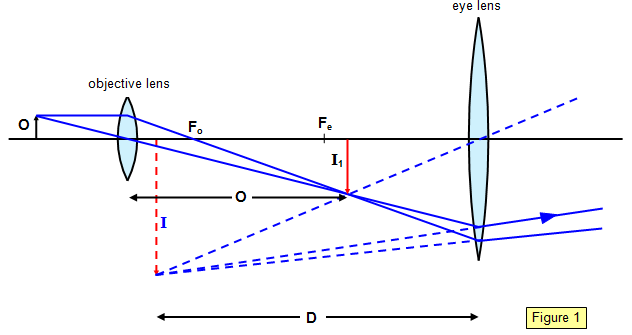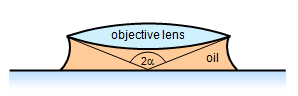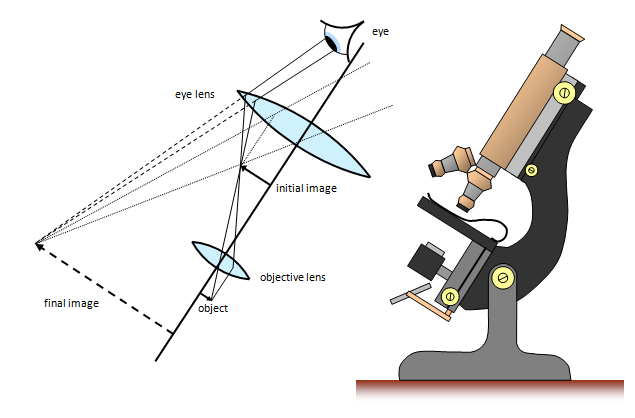# The compound microscope

The compound microscope (Figure 1) uses several lenses to produce a highly magnified image of an object.The diagram shows the microscope in normal adjustment, that is, with the final image at the near point (25 cm from the eye) (distance D from the eye lens). This setting gives the maximum angular size of image without eye strain.

The objective lens produces a real, inverted image of the object 0 at I1 This is then viewed by the eye lens and this gives a final virtual image at I. The magnifying power M of the instrument is given by the formula:

Magnifying power (M) = [D/fe – 1][v/fo – 1]

where fe is the focal length of the eye lens, fo that of the objective lens and v the distance of I1 from the objective lens.

Therefore you can see that the smaller the focal lengths of the objective and eye lens, the greater is the magnifying power. If the focal length of the objective is too small, however, the object will subtend too great an angle with it and the image will be distorted due to off-axis rays. This is the reason for using the oil immersion objective to reduce the amount of refraction at the first lens surface.Resolving power of microscope = λ/[2nsin(α)]

The resolving power of a microscope can be shown to depend on the wavelength of light used (λ), the refractive index of the medium above the slide (n) and the angle subtended at the objective (α) (Figure 2):

An alternative and very useful formula for the magnifying power M of a compound microscope is:

Magnifying power (M) = mo x me

where mo and me are the magnifications of the objective lens and eyepiece lens respectively.

1. Two thin convex lenses are used to form a compound microscope. An object 3 cm in front of the objective lens gives a final image at the same position as the object and 25 cm from the eye lens, the magnification being 15.
Calculate the focal lengths of both the objective lens and the eye lens.

2. A compound microscope is formed from two convex lenses, an eyepiece of focal length 5.0 cm and an objective of focal length 1.0 cm. If an object is placed 1.1 cm from the objective, the final image is formed 32.0 cm from the eye lens.
Calculate the magnifying power of the instrument if the near point is 25 cm from the observer's eye.A VERSION IN WORD IS AVAILABLE ON THE SCHOOLPHYSICS USB